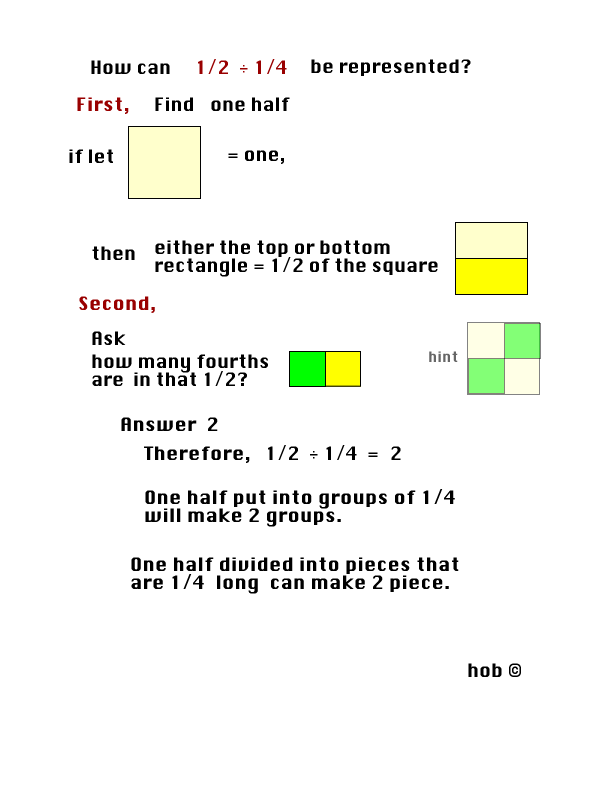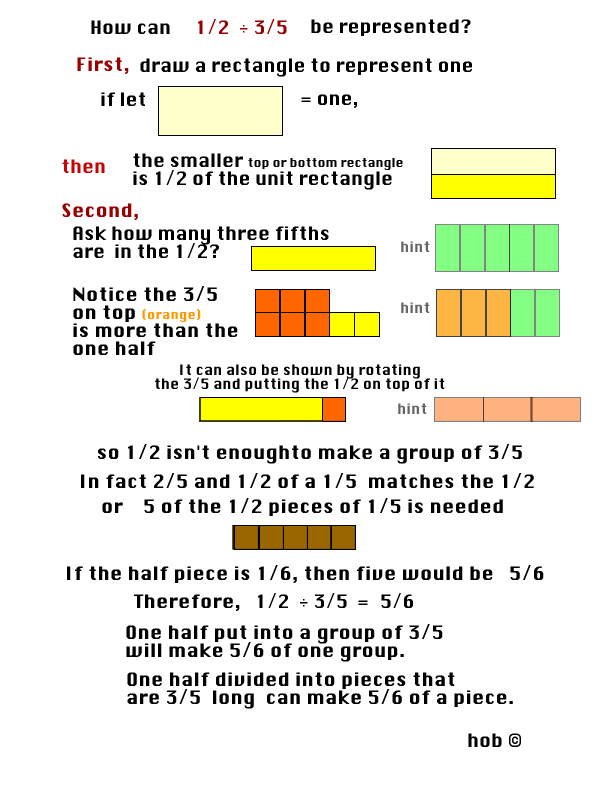# Division of fractions representations

## One-half divided by one half represented with squares and rectangles## One-half divided by one-fourth represented with squares and rectangles## One-half divided by three-fourths represented with squares and rectangles## One-half divided by three-fifths represented with squares and rectangles

Thanks BrantDiscussion:When these representations are understood begin to connect to decimals.

Mind boggler:

• How many dimes in \$2.00?
• 2 ÷ 1/10 = 20 Draw a representation.

Enjoy!